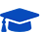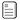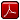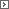Mathematics Adult High School Completion 1-2Course PrefacePrintable Version (pdf)Course Introduction

Core Standards of the Course

Standards for Mathematical Practice

MP.1
Make sense of problems and persevere in solving them.
Mathematically proficient students start by explaining to themselves the meaning of a problem and looking for entry points to its solution. They analyze givens, constraints, relationships, and goals. They make conjectures about the form and meaning of the solution and plan a solution pathway rather than simply jumping into a solution attempt. They consider analogous problems, and try special cases and simpler forms of the original problem in order to gain insight into its solution. They monitor and evaluate their progress and change course if necessary. Students might, depending on the context of the problem, transform algebraic expressions or change the viewing window on their graphing calculator to get the information they need. Mathematically proficient students can explain correspondences between equations, verbal descriptions, tables, and graphs or draw diagrams of important features and relationships, graph data, and search for regularity or trends. Less experienced students might rely on using concrete objects or pictures to help conceptualize and solve a problem. Mathematically proficient students check their answers to problems using a different method, and they continually ask themselves, "Does this make sense?" They can understand the approaches of others to solving complex problems and identify correspondences between different approaches.

MP.2
Reason abstractly and quantitatively.
Mathematically proficient students make sense of quantities and their relationships in problem situations. They bring two complementary abilities to bear on problems involving quantitative relationships: the ability to decontextualize-to abstract a given situation and represent it symbolically and manipulate the representing symbols as if they have a life of their own, without necessarily attending to their referents-and the ability to contextualize, to pause as needed during the manipulation process in order to probe into the referents for the symbols involved. Quantitative reasoning entails habits of creating a coherent representation of the problem at hand; considering the units involved; attending to the meaning of quantities, not just how to compute them; and knowing and flexibly using different properties of operations and objects.

MP.3
Construct viable arguments and critique the reasoning of others.
Mathematically proficient students understand and use stated assumptions, definitions, and previously established results in constructing arguments. They make conjectures and build a logical progression of statements to explore the truth of their conjectures. They are able to analyze situations by breaking them into cases, and can recognize and use counterexamples. They justify their conclusions, communicate them to others, and respond to the arguments of others. They reason inductively about data, making plausible arguments that take into account the context from which the data arose. Mathematically proficient students are also able to compare the effectiveness of two plausible arguments, distinguish correct logic or reasoning from that which is flawed, and-if there is a flaw in an argument-explain what it is. Less experienced students can construct arguments using concrete referents such as objects, drawings, diagrams, and actions. Such arguments can make sense and be correct, even though they are not generalized or made formal until later. Later, students learn determine domains to which an argument applies. Students at all levels can listen or read the arguments of others, decide whether they make sense, and ask useful questions to clarify or improve the arguments.

MP.4
Model with mathematics.
Mathematically proficient students can apply the mathematics they know to solve problems arising in everyday life, society, and the workplace. This might be as simple as writing an addition equation to describe a situation. A student might apply proportional reasoning to plan a school event or analyze a problem in the community. A student might use geometry to solve a design problem or use a function to describe how one quantity of interest depends on another. Mathematically proficient students who can apply what they know are comfortable making assumptions and approximations to simplify a complicated situation, realizing that these may need revision later. They are able to identify important quantities in a practical situation and map their relationships using such tools as diagrams, two-way tables, graphs, flowcharts and formulas. They can analyze those relationships mathematically to draw conclusions. They routinely interpret their mathematical results in the context of the situation and reflect on whether the results make sense, possibly improving the model if it has not served its purpose.

MP.5
Use appropriate tools strategically.
Mathematically proficient students consider the available tools when solving a mathematical problem. These tools might include pencil and paper, concrete models, a ruler, a protractor, a calculator, a spreadsheet, a computer algebra system, a statistical package, or dynamic geometry software. Proficient students are sufficiently familiar with tools appropriate for their course to make sound decisions about when each of these tools might be helpful, recognizing both the insight to be gained and their limitations. For example, mathematically proficient students analyze graphs of functions and solutions generated using a graphing calculator. They detect possible errors by strategically using estimation and other mathematical knowledge. When making mathematical models, they know that technology can enable them to visualize the results of varying assumptions, explore consequences, and compare predictions with data. Mathematically proficient students at various levels are able to identify relevant external mathematical resources, such as digital content located on a website, and use them to pose or solve problems. They are able to use technological tools to explore and deepen their understanding of concepts.

MP.6
Attend to precision.
Mathematically proficient students try to communicate precisely to others. They try to use clear definitions in discussion with others and in their own reasoning. They state the meaning of the symbols they choose, including using the equal sign consistently and appropriately. They are careful about specifying units of measure, and labeling axes to clarify the correspondence with quantities in a problem. They calculate accurately and efficiently, express numerical answers with a degree of precision appropriate for the problem context. Less experienced students give carefully formulated explanations to each other. By the time they reach high school they have learned to examine claims and make explicit use of definitions.

MP.7
Look for and make use of structure.
Mathematically proficient students look closely to discern a pattern or structure. Students, for example, might notice that three and seven more is the same amount as seven and three more, or they may sort a collection of shapes according to how many sides the shapes have. Later, students will see 7 � 8 equals the well-remembered 7 � 5 + 7 � 3, in preparation for learning about the distributive property. In the expression x2+ 9x + 14, students can see the 14 as 2 � 7 and the 9 as 2 + 7. They recognize the significance of an existing line in a geometric figure and can use the strategy of drawing an auxiliary line for solving problems. They also can step back for an overview and shift perspective. They can see complicated things, such as some algebraic expressions, as single objects or as being composed of several objects. For example, they can see 5 - 3(x - y)2 as 5 minus a positive number times a square and use that to realize that its value cannot be more than 5 for any real numbers x and y.

MP.8
Look for and express regularity in repeated reasoning.
Mathematically proficient students notice if calculations are repeated, and look both for general methods and for shortcuts. Early on, students might notice when dividing 25 by 11 that they are repeating the same calculations over and over again, and conclude they have a repeating decimal. By paying attention to the calculation of slope as they repeatedly check whether points are on the line through (1, 2) with slope 3, students might abstract the equation (y - 2)/(x - 1) = 3. Noticing the regularity in the way terms cancel when expanding (x-1)(x+1), (x-1)(x2+x+1), and (x-1)(x3 +x2+x+1) might lead them to the general formula for the sum of a geometric series. As they work to solve a problem, mathematically proficient students maintain oversight of the process, while attending to the details. They continually evaluate the reasonableness of their intermediate results.

Number and Quantity: The Real Number System

Extend the properties of exponents to rational exponents.

N.RN.2
Rewrite expressions involving radicals and rational exponents using the properties of exponents.

Number and Quantity: Quantities

Reason quantitatively and use units to solve problems.

N.Q.1
Use units as a way to understand problems and to guide the solution of multi-step problems; choose and interpret units consistently in formulas; choose and interpret the scale and the origin in graphs and data displays.*

N.Q.3
Choose a level of accuracy appropriate to limitations on measurement when reporting quantities.* [Also see 8.EE.4]

Algebra: Seeing Structure in Expressions

Interpret the structure of expressions.

A.SSE.1
Interpret expressions that represent a quantity in terms of its context.*

1. Interpret parts of an expression, such as terms, factors, and coefficients.* (A.SSE.1a)

A.SSE.2
Use the structure of an expression to identify ways to rewrite it. For example, see x4 - y4 as (x2)2 - (y2)2, thus recognizing it as a difference of squares that can be factored as (x2 - y2)(x2 + y2). [Also see 7.EE.2]

Write expressions in equivalent forms to solve problems.

A.SSE.3
Choose and produce an equivalent form of an expression to reveal and explain properties of the quantity represented by the expression.* [Also see 7.EE.2]

1. Factor a quadratic expression to reveal the zeros of the function it defines.* (A.SSE.3a) [Also see 7.EE.2]

Algebra: Arithmetic with Polynomials and Rational Expressions

Perform arithmetic operations on polynomials.

A.APR.1
Understand that polynomials form a system analogous to the integers, namely, they are closed under the operations of addition, subtraction, and multiplication; add, subtract, and multiply polynomials. [Note from panel: Emphasis should be on operations with polynomials.]

Rewrite rational expressions.

A.APR.6
Rewrite simple rational expressions in different forms; write a(x)/b(x) in the form q(x) + r(x)/b(x), where a(x), b(x), q(x), and r(x) are polynomials with the degree of r(x) less than the degree of b(x), using inspection, long division, or, for the more complicated examples, a computer algebra system.

Algebra: Creating Equations

Create equations that describe numbers or relationships.

A.CED.1
Create equations and inequalities in one variable and use them to solve problems. Include equations arising from linear and quadratic functions, and simple rational and exponential functions.* [Also see 7.EE.4, 7.EE.4a, and 7.EE.4b]

A.CED.2
Create equations in two or more variables to represent relationships between quantities; graph equations on coordinate axes with labels and scales.*

A.CED.3
Represent constraints by equations or inequalities, and by systems of equations and/or inequalities, and interpret solutions as viable or non-viable options in a modeling context. For example, represent inequalities describing nutritional and cost constraints on combinations of different foods.*

A.CED.4
Rearrange formulas to highlight a quantity of interest, using the same reasoning as in solving equations. For example, rearrange Ohm's law V = IR to highlight resistance R.* [Also see 7.EE.2]

Algebra: Reasoning with Equations and Inequalities

Understand solving equations as a process of reasoning and explain the reasoning.

A.REI.1
Explain each step in solving a simple equation as following from the equality of numbers asserted at the previous step, starting from the assumption that the original equation has a solution. Construct a viable argument to justify a solution method.

A.REI.2
Solve simple rational and radical equations in one variable, and give examples showing how extraneous solutions may arise. [Also see 8.EE.2]

Solve equations and inequalities in one variable.

A.REI.3
Solve linear equations and inequalities in one variable, including equations with coefficients represented by letters. [Also see 7.EE.4, 7.EE.4a, 7.EE.4b, and 8.EE.7]

A.REI.4
Solve quadratic equations in one variable.

Solve systems of equations.

A.REI.6
Solve systems of linear equations exactly and approximately (e.g., with graphs), focusing on pairs of linear equations in two variables. [Also see 8.EE.8b]

Represent and solve equations and inequalities graphically.

A.REI.10
Understand that the graph of an equation in two variables is the set of all its solutions plotted in the coordinate plane, often forming a curve (which could be a line). [Also see 8.F.5]

Functions: Interpreting Functions

Understand the concept of a function and use function notation.

F.IF.1
Understand that a function from one set (called the domain) to another set (called the range) assigns to each element of the domain exactly one element of the range. If f is a function and x is an element of its domain, then f(x) denotes the output of f corresponding to the input x. The graph of f is the graph of the equation y = f(x). [Also see 8.F.1]

F.IF.2
Use function notation, evaluate functions for inputs in their domains, and interpret statements that use function notation in terms of a context.

Interpret functions that arise in applications in terms of the context.

F.IF.4
For a function that models a relationship between two quantities, interpret key features of graphs and tables in terms of the quantities, and sketch graphs showing key features given a verbal description of the relationship. For example, for a quadratic function modeling a projectile in motion, interpret the intercepts and the vertex of the function in the context of the problem.* [Key features include: intercepts; intervals where the function is increasing, decreasing, positive, or negative; relative maximums and minimums; symmetries; end behavior; and periodicity.]

F.IF.5
Relate the domain of a function to its graph and, where applicable, to the quantitative relationship it describes. For example, if the function h(n) gives the number of person-hours it takes to assemble n engines in a factory, then the positive integers would be an appropriate domain for the function.*

F.IF.6
Calculate and interpret the average rate of change of a function (presented symbolically or as a table) over a specified interval. Estimate the rate of change from a graph.* [NOTE: See conceptual modeling categories.]

Analyze functions using different representations.

F.IF.7
Graph functions expressed symbolically and show key features of the graph, by hand in simple cases and using technology for more complicated cases.* [Also see 8.F.5]

F.IF.8b
Use properties of exponents to interpret expressions for exponential functions. For example, identify percent rate of change in an exponential function and then classify it as representing exponential growth or decay. [Also see 8.EE.1]

F.IF.9
Compare properties of two functions each represented in a different way (algebraically, graphically, numerically in tables, or by verbal descriptions). For example, given a linear function represented by a table of values and a linear function represented by an algebraic expression, determine which function has the greater rate of change.

Functions: Building Functions

Build a function that models a relationship between two quantities.

F.BF.1
Write a function that describes a relationship between two quantities.* [Also see 8.F.4]

Functions: Linear, Quadratic, and Exponential Models

Construct and compare linear, quadratic, and exponential models and solve problems.

F.LE.1
Distinguish between situations that can be modeled with linear functions and with exponential functions.*

1. Recognize situations in which one quantity changes at a constant rate per unit interval relative to another.* (F.LE.1b)
2. Recognize situations in which a quantity grows or decays by a constant percent rate per unit interval relative to another.* (F.LE.1c)

Interpret expressions for functions in terms of the situation they model.

F.LE.5
Interpret the parameters in a linear or exponential function in terms of a context.* [Also see 8.F.4]

Geometry: Congruence

Experiment with transformations in the plane.

G.CO.1
Know precise definitions of angle, circle, perpendicular line, parallel line, and line segment, based on the undefined notions of point, line, distance along a line, and distance around a circular arc.

Geometry: Similarity, Right Triangles, and Trigonometry

Prove theorems involving similarity.

G.SRT.5
Use congruence and similarity criteria for triangles to solve problems and to prove relationships in geometric figures. [Also see 8.G.2 and 8.G.4]

Geometry: Geometric Measurement and Dimension

Explain volume formulas and use them to solve problems.

G.GMD.3
Use volume formulas for cylinders, pyramids, cones, and spheres to solve problems.* [Also see 7.G.6]

Geometry: Modeling with Geometry

Apply geometric concepts in modeling situations.

G.MG.2
Apply concepts of density based on area and volume in modeling situations (e.g., persons per square mile, BTUs per cubic foot).* [Also see 7.RP.3]

Statistics and Probability: Interpreting Categorical and Quantitative Data

Summarize, represent, and interpret data on a single count or measurable variable.

S.ID.1
Represent data with plots on the real number line (dot plots, histograms, and box plots). [Also see 6.SP.4 and 8.SP.1]

S.ID.3
Interpret differences in shape, center, and spread in the context of the data sets, accounting for possible effects of extreme data points (outliers). [Also see 7.SP.4]

Summarize, represent, and interpret data on two categorical and quantitative variables.

S.ID.5
Summarize categorical data for two categories in two-way frequency tables. Interpret relative frequencies in the context of the data (including joint, marginal, and conditional relative frequencies). Recognize possible associations and trends in the data. [Also see 8.SP.4]

Interpret linear models.

S.ID.7
Interpret the slope (rate of change) and the intercept (constant term) of a linear model in the context of the data. [Also see 8.SP.3]

S.ID.9
Distinguish between correlation and causation.http://www.uen.org - in partnership with Utah State Board of Education (USBE) and Utah System of Higher Education (USHE).  Send questions or comments to USBE Specialist - BRIAN  OLMSTEAD and see the Adult Ed/ Mathematics website. For general questions about Utah's Core Standards contact the Director - BRIAN  OLMSTEAD.

These materials have been produced by and for the teachers of the State of Utah. Copies of these materials may be freely reproduced for teacher and classroom use. When distributing these materials, credit should be given to Utah State Board of Education. These materials may not be published, in whole or part, or in any other format, without the written permission of the Utah State Board of Education, 250 East 500 South, PO Box 144200, Salt Lake City, Utah 84114-4200.